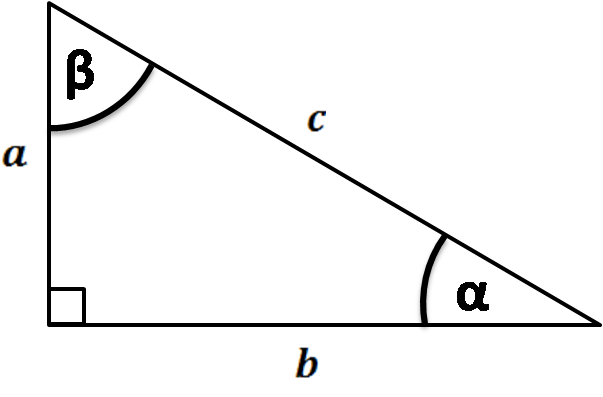# Sine Triangle Calculator

Created by Arturo Barrantes
Reviewed by Dominika Miszewska, MD, PhD candidate
Last updated: Jun 09, 2022

The handy sine triangle calculator is a tool that finds out the sine of an angle that can be expressed in degrees, radians, or pi radians. In this article, we will explain what the sine is, how to calculate it, and will show several examples.

## What is the sine in Trigonometry?

The sine of an angle is a trigonometric function that relates two triangle sides: The opposite side to the angle and the hypotenuse. Of course, it's better to explain with a picture:As you can see, $\beta$ is the angle with $b$ as a triangle opposite side. Then, the sine of $\beta$ is the division between $c$ and the hypotenuse, $c$. In other words, the sine formula is:

$sin (\beta) = \frac{b}{c}.$

## How to calculate the sine of an angle?

You can use our sine triangle calculator or do the following:

1. Pick the opposite triangle side of the angle you are interested in. In the above picture, we have represented the opposite triangle side of $\beta$ as $b$. See that $a$ would correspond to $\alpha$
2. Divide $b$ by $c$ for getting the sine of $\beta$.

## Sine triangle example - What is the sine of 30 degrees?

It is 0.5. How? Try Omnicalculator's sine triangle calculator or do the following:

1. Notice you have a 30-60 right triangle. This one is special because all the sides are in constant relationship. The hypotenuse is equivalent to 2; meanwhile, the other sides are equivalent to 1 and $\sqrt{3}$
2. The opposite side of the 30 degrees angle is the equivalent to 1. Therefore, we divide 1 by 2 and get 0.5.

## Other useful tools like the sine triangle calculator

Since you already know how to calculate the sine of an angle, you can take a look at other calculators:

## FAQ

### How do you get the sine of an angle?

Try Omnicalculator's sine triangle calculator or do the following:

1. Find the opposite side of the angle you are interested in.
2. Divide such opposite side by the hypotenuse of the triangle.
3. Confirm the result is not over 1. Congratulations, you have calculated the sine of an angle.

### What is the sine of 60 degrees?

0.86. You can verify it with Omnicalculator's sine triangle calculator or:

1. Recognize you have a 30-60 right triangle where the opposite side of your triangle is equivalent to the square root of 3.
2. Divide such side by the hypotenuse, which is equivalent to 2. The result is the sine of 60 degrees.

### What is the sine of 45 degrees?

0.71. You can verify it with Omnicalculator's sine triangle calculator or:

1. Recognize you have a 45-45 right triangle where the opposite side of your triangle is equivalent to 1.
2. Divide such side by the hypotenuse, which is equivalent to the square root of 2. The result is the sine of 45 degrees.
Arturo Barrantes
Trigonometric functions: sin, cos, tan...
Angle
deg
People also viewed…

### Body fat

Use the body fat calculator to estimate what percentage of your body weight comprises of body fat.

### Car crash force

With this car crash calculator, you can find out how dangerous are car crashes.

### Sector area

Sector area calculator can find the sector area of a circle in the blink of an eye.

### Trigonometric functions

Trigonometric functions calculator will find the sine, cosine, tangent, cotangent, secant, and cosecant of any chosen angle. Enter the angle value in degrees or radians, and find all essential trigonometric functions.